# Definition

A `line chart` or line graph displays the evolution of one or several numeric variables. Data points are connected by straight line segments. It is similar to a scatter plot except that the measurement points are ordered (typically by their x-axis value) and joined with straight line segments. A line chart is often used to visualize a trend in data over intervals of time – a time series – thus the line is often drawn chronologically.

The following example shows the evolution of the bitcoin price between April 2013 and April 2018. Data comes from the CoinMarketCap website.

``````# Libraries
library(tidyverse)
library(hrbrthemes)
library(plotly)
library(patchwork)
library(babynames)
library(viridis)

data\$date <- as.Date(data\$date)

# plot
data %>%
ggplot( aes(x=date, y=value)) +
geom_line(color="#69b3a2") +
ggtitle("Evolution of Bitcoin price") +
ylab("bitcoin price (\$)") +
theme_ipsum()``````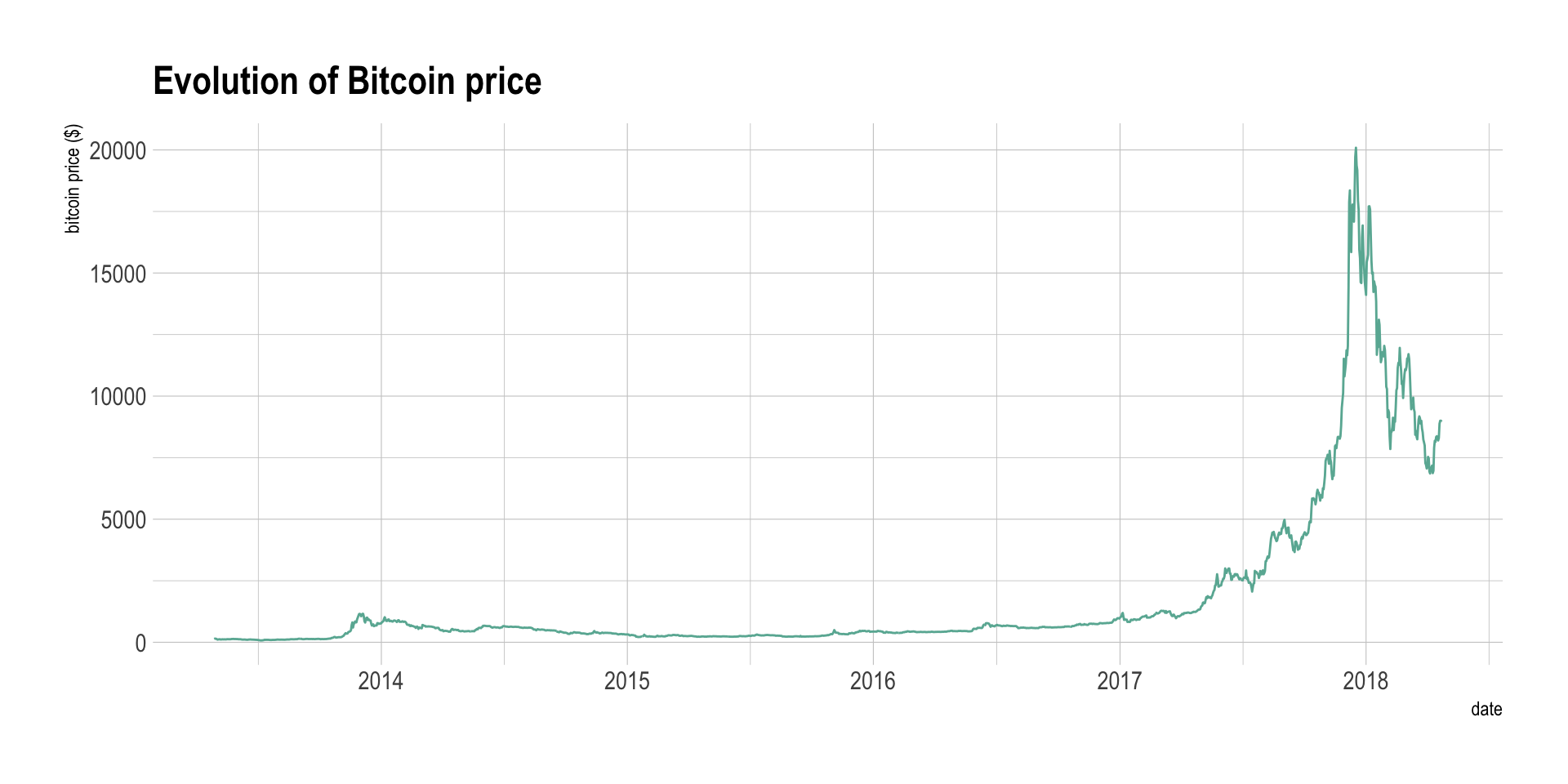# What for

Line chart can be used to show the evolution of one (like above) or several variables. Here is an example showing the evolution of three baby name frequencies in the US between 1880 and 2015. Note that this works well for a low number of group to display. With more than a few, the graphic get cluttered and becomes unreadable. This is called a spaghetti chart and you can read more about it here.

``````# Load dataset from github
don <- babynames %>%
filter(name %in% c("Ashley", "Patricia", "Helen")) %>%
filter(sex=="F")

# Plot
don %>%
ggplot( aes(x=year, y=n, group=name, color=name)) +
geom_line() +
scale_color_viridis(discrete = TRUE) +
ggtitle("Popularity of American names in the previous 30 years") +
theme_ipsum() +
ylab("Number of babies born")``````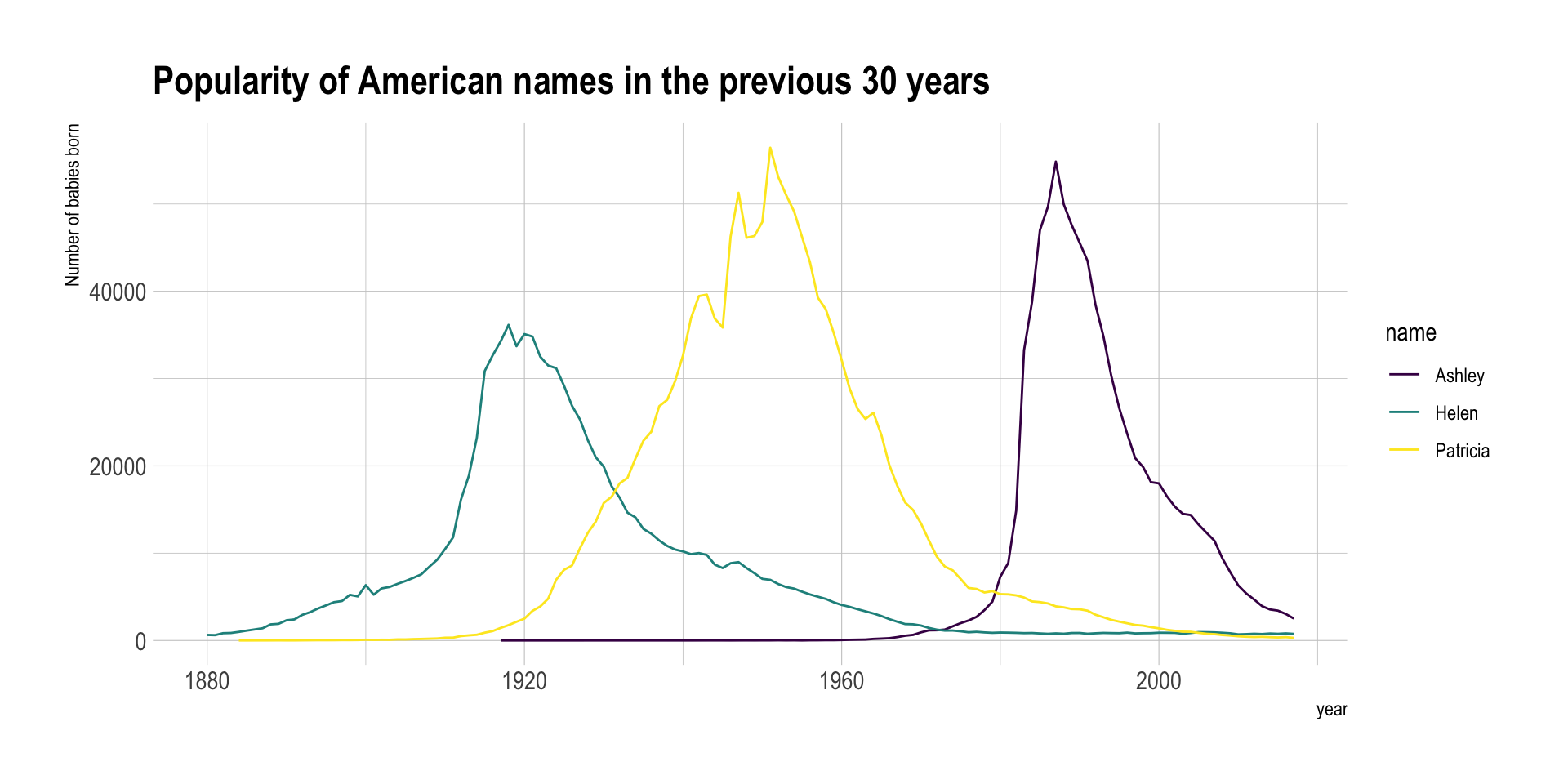# Variation

If the number of data points is low, it is advised to represent each individual observation with a dot. It allows to understand when exactly the observation have been made:

``````data %>%
tail(10) %>%
ggplot( aes(x=date, y=value)) +
geom_line(color="#69b3a2") +
geom_point(color="#69b3a2", size=4) +
ggtitle("Cuting") +
ylab("bitcoin price (\$)") +
theme_ipsum()``````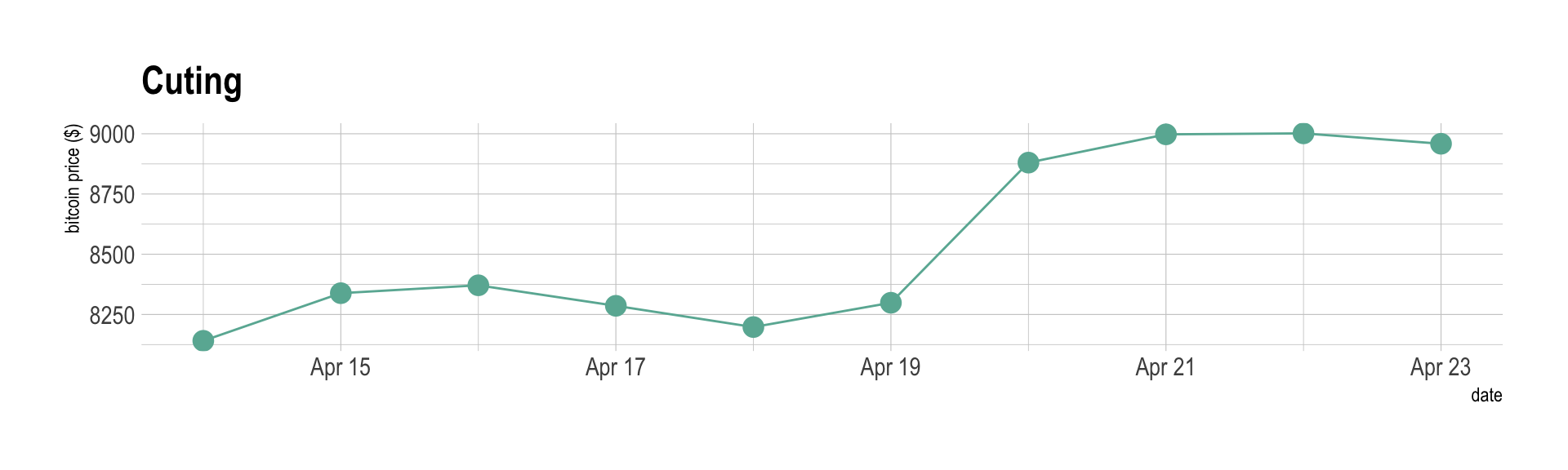Note that lines are also used to show trends in a scatterplot. Here is an example using Smoothed conditional means and showing confidence interval around it:

``````ggplot(mpg, aes(displ, hwy)) +
geom_point() +
geom_smooth(color="#69b3a2") +
theme_ipsum()``````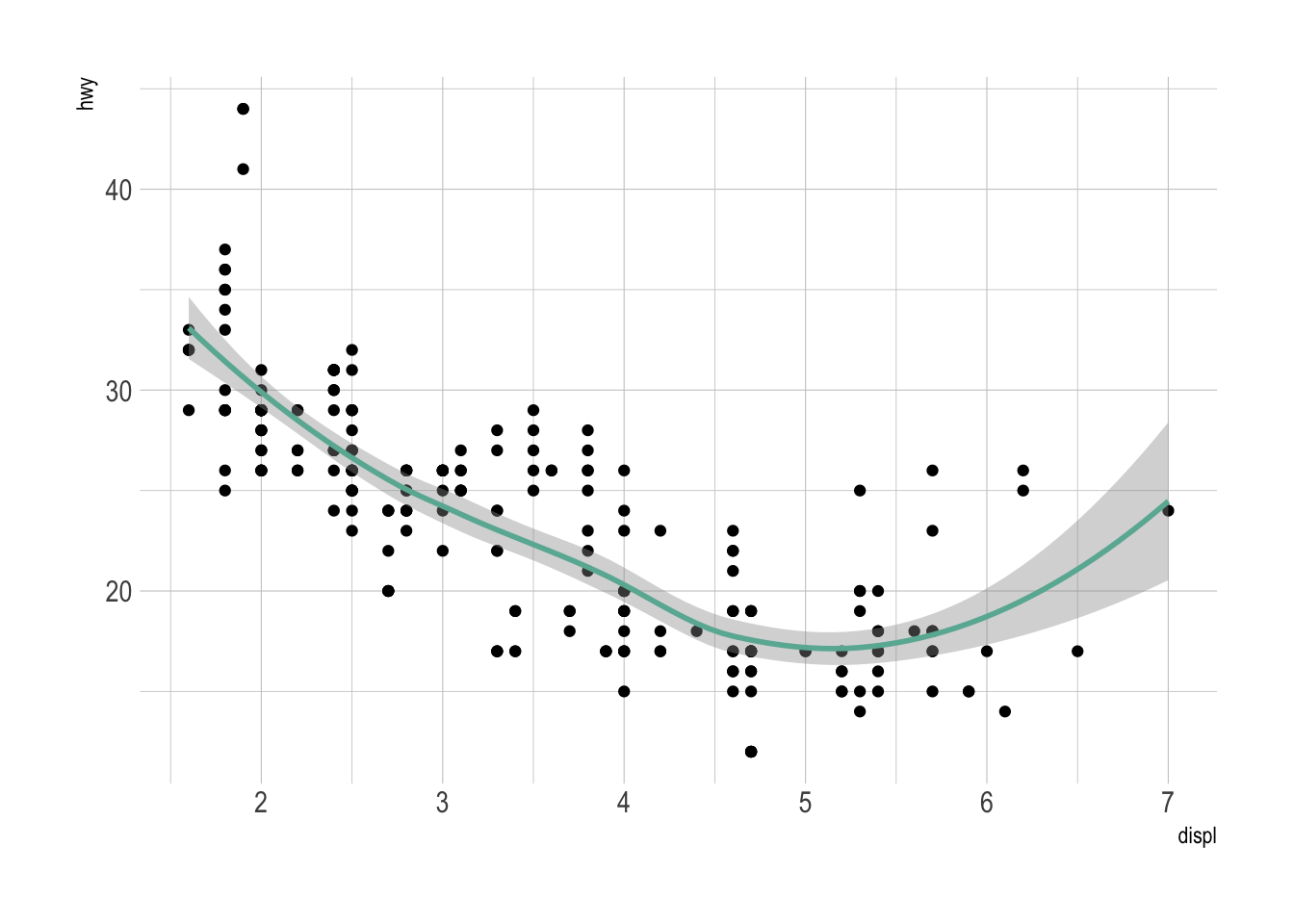Note: this example comes from the ggplot2 documentaion.

# Common caveats

The line chart is subject to a lot of discussion concerning potential caveats. Here is an overview:

• To cut or not to cut the Y axis? Wether or not the Y axis must start at 0 is a hot topic leading to intense debates. The graphic below presents the same data, starting at 0 (left) or not (right). Generally, line plot do not need to start at 0 since it allows to observe patterns more efficiently, but you probably want to learn more about it here.
``````p1 <- data %>%
tail(10) %>%
ggplot( aes(x=date, y=value)) +
geom_line(color="#69b3a2") +
geom_point(color="#69b3a2", size=4) +
ggtitle("Not cuting") +
ylab("bitcoin price (\$)") +
theme_ipsum() +
ylim(0,10000)

p2 <- data %>%
tail(10) %>%
ggplot( aes(x=date, y=value)) +
geom_line(color="#69b3a2") +
geom_point(color="#69b3a2", size=4) +
ggtitle("Cuting") +
ylab("bitcoin price (\$)") +
theme_ipsum()

p1 + p2``````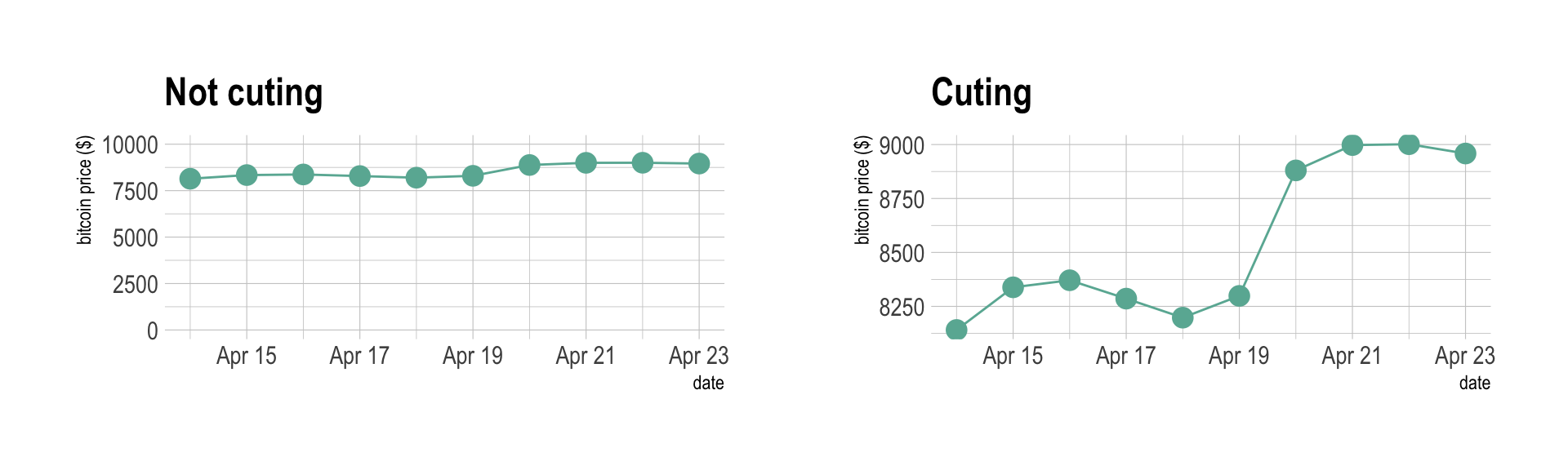• If you need to compare the evolution of 2 different variables, do not use dual axis. Indeed dual axis can show very different results depending on what range you apply to the axis. Read more about it.
• Mind the spaghetti chart: too many lines make the chart unreadable.
• Think about the aspect ratio of the graphic, extreme ratio make the chart unreadable.

# Related

The R and Python graph galleries are 2 websites providing hundreds of chart example, always providing the reproducible code. Click the button below to see how to build the chart you need with your favorite programing language.